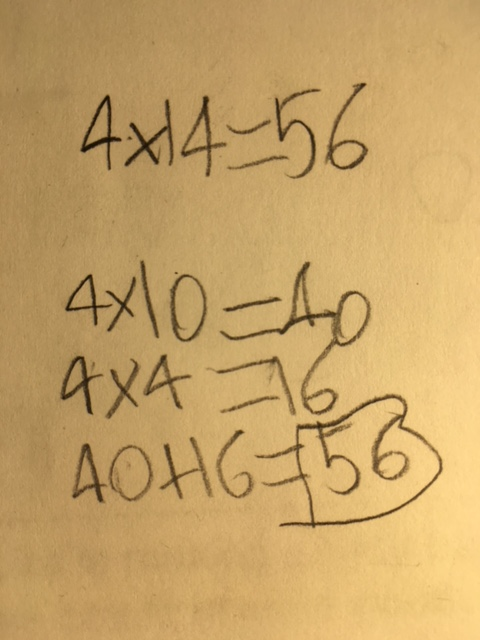#### PS/IS 686 | Brooklyn, NY

Division is wrapping up in third grade, so I wanted to share some of the students’ division strategies with you. It’s interesting seeing all the different ways there are to  break a total into equal groups – and children who are able to reason in these ways are showing that they truly understand what’s happening when you work with equal groups.

The way a number of third graders initially approached division was by repeated addition or skip counting. If we have 24 candies and we need to put 4 in each bag, then to find out how many bags we will need, we can visualize bagging up 4 candies at a time: 4, 8, 12, 16, 20, 24. Then we count how many “bags” we made, and see that it’s 6. Alternatively, we could start with 24 and subtract 4 at a time, over and over, until the candies are all gone: 20, 16, 12, 8, 4, 0. These strategies show that a child is visualizing and that he or she understands that equal groups are involved, so it’s completely appropriate to see them when we’re first exploring division. However, they’re actually more additive than multiplicative, and as our unit has progressed, I expect to see children starting to reason about chunks of equal groups. The below strategies are all flexible and intelligent responses to the challenge of making equal groups, and they all arose from the students’ active search for ways to solve division problems.

A classic strategy for dividing by 4 is to find half, and then find half again. As the students pointed out during our Congress on division strategies, halving will also work if you’re trying to divide by 8 or 16.“To divide by 4, find half, and then half again” (Mia)

“Dealing out” is a strategy that my own family used when sharing something like a big bowl of cherries. If you “deal out by 1s” you go around and around giving each person 1 at a time until the total is all gone. That can take a very long time, though! “Dealing out by chunks” is much quicker. Here, Grace knew that she could easily give each person 10. Then she calculated that she had 16 left. When she tried giving each person 4, it worked out perfectly! One strategy for dealing out in chunks is to use numbers that it’s easy to skip count by: 10, 5, or 2. The main challenge is that you have to keep careful track of how many you have left to give out.“Dealing out” (Grace)

Many of the students approach division as a “missing factor” problem. Instead of thinking 56 divided by 4, they think 4 x X = 56. This is akin to solving subtraction by using addition: solving 256 – 81 as 81 + X = 256. It shows that they understand that multiplication and division are inverse operations. It’s also usually easier to think about multiplication than division!

Once you’re thinking 4 x X = 56, then what? Aleksander started with 10 x 4. Why? Because in third grade language, it’s “friendly”. He knew that 4×4 = 16, and he saw that 40 + 16 would equal 56.Brianna started with 11×4 = 44, and then she kept careful track of her thinking as she skip counted up from there. This is kind of like taking the express train, and then changing to the local till you get to your stop.Ten, and multiples of 10, are “friendly” numbers to work with. Chiara thought, “Well, I don’t know what 56 divided by 4 is, but I do know what 40 divided by 4 is!” What she was doing was decomposing the total into friendlier numbers, numbers that a student finds it easier to reason about.“Decompose the total into friendlier numbers” (Chiara)

Chiara was looking for ways that she could reason using the facts she knew. This general strategy, using what you know to figure out what you don’t yet know, is tremendously useful. To solve 42 divided by 6, Eli started with 6×10 = 60, and then reasoned from there by subtracting equal groups. It’s small in the image, but his bottom line reads 60 – 18 = 42, and under the 18, he wrote 3 x 6. Another way to show his reasoning would be to write (10 x 6) – (3 x 6) = 7 x 6.“Reason using the facts you know” (Eli)

The last approach isn’t a strategy; we really consider it a tool for organizing your thinking. The ratio table is a wonderful tool, though, which can be used for multiplication or, when you know the number of items in each group, for division as well. The basic principle in using a ratio table is that whatever you do to one row (goody bags) you must also do to the other row (candies in each bag). If you add one bag, then you must add the number of candies in one bag. If you double, or triple, the number of bags, then you must also double or triple the number of candies. (At first, students just used ratio tables to organize and present their skip counting – just adding on one group each time – but once you start doubling, it become much more efficient.) Here, Hazel knew that she needed to figure out the number of bags that would hold 42 candies, so she thought carefully about when to double and when to add single bags so that she could find the number of bags that went with 42 candies.“If you know the number in each group, use a ratio table” (Hazel)